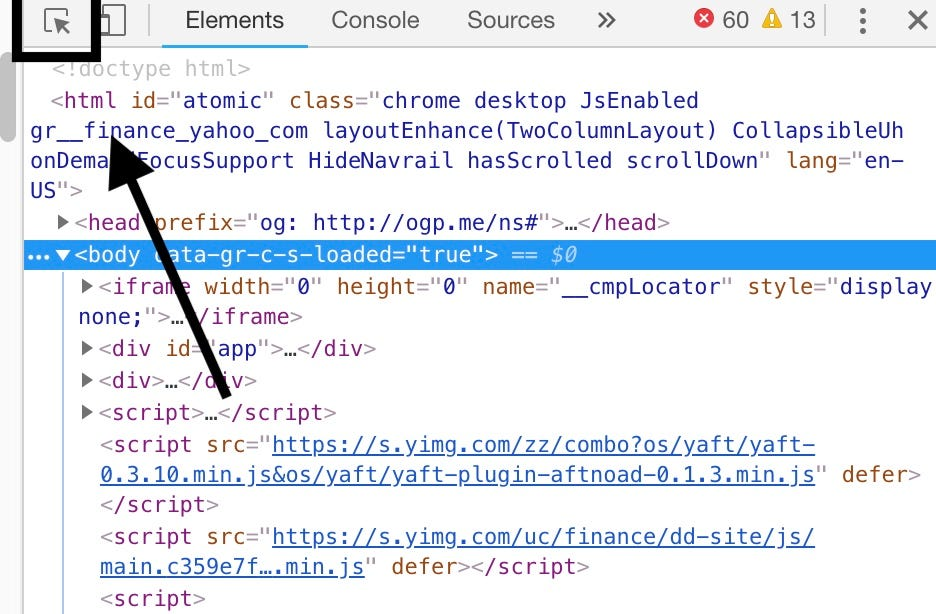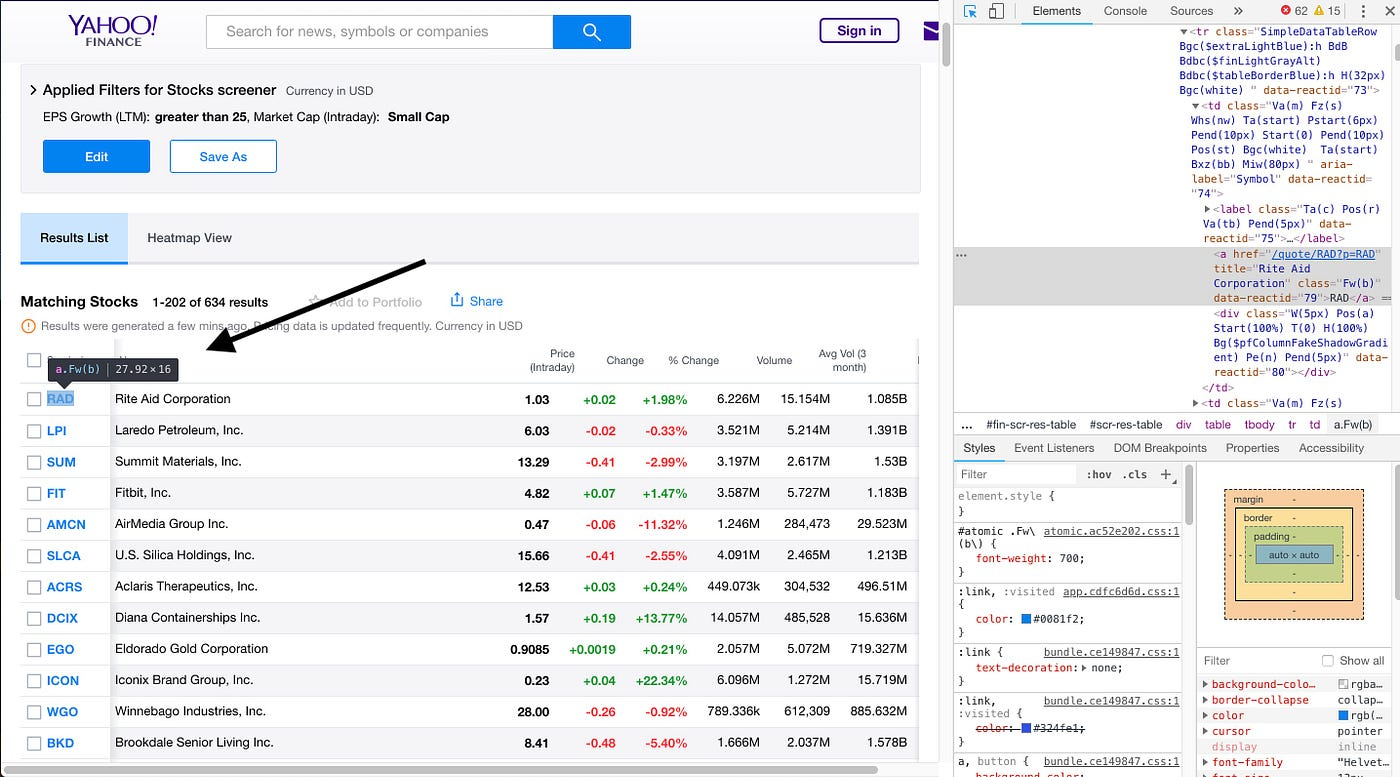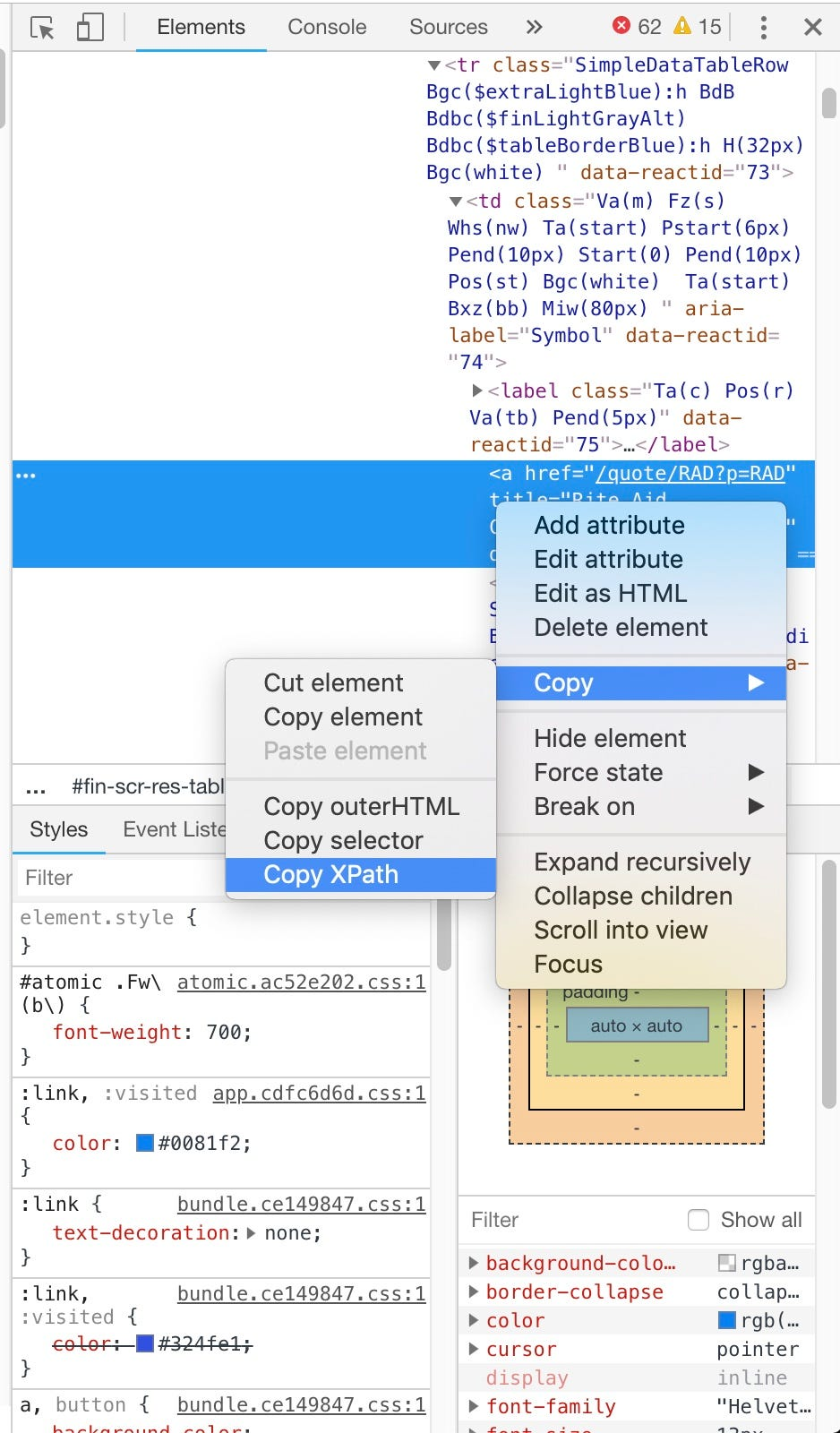# Predicting Stock Prices with PythonPredicting Stock Prices with Python .Predicting stock prices from Yahoo stock screener using scikit-learn and sending the predicitons via smtplib to a phone number. Time series analysis (Linear Regression) is used, sciki-learn, to predict the future prices from the stock tickers.

Investing in the stock market used to require a ton of capital and a broker that would take a cut from your earnings. Then Robinhood disrupted the industry allowing you to invest as little as \$1 and avoid a broker altogether. Robinhood and apps like it have opened up investing to anyone with a connected device and gave non-investors the opportunity to profit from the newest tech start-up.

However, giving those of us who are not economists or accountants the freedom to invest our money in the “hottest” or “trending” stocks is not always the best financial decision.

Thousands of companies use software to predict the movement in the stock market in order to aid their investing decisions. The average Robinhood user does not have this available to them. Primitive predicting algorithms such as a time-sereis linear regression can be done with a time series prediction by leveraging python packages like scikit-learn and iexfinnance.

This program will scrape a given amount of stocks from the web, predict their price in a set number of days and send an SMS message to the user informing them of stocks that might be good to check out and invest in.

## Setup

In order to create a program that predicts the value of a stock in a set amount of days, we need to use some very useful python packages. You will need to install the following packages:

If you do not already have some of these packages you can install them through `pip install PACKAGE` or by cloning the git repository.

Here is an example of installing numpy with pip

``````pip install numpy
``````

and with git

``````git clone https://github.com/numpy/numpy
cd numpy
python setup.py install
``````

Now open up your favorite text editor and create a new python file. Start by importing the following packages

``````import numpy as np
from datetime import datetime
import smtplib
import time
from selenium import webdriver
#For Prediction
from sklearn.linear_model import LinearRegression
from sklearn import preprocessing, cross_validation, svm
#For Stock Data
from iexfinance import Stock
from iexfinance import get_historical_data
``````

Note: the datetime, time and smtplib packages come with python

In order to scrape the Yahoo stock screener, you will also need to install the Chromedriver in order to properly use Selenium. That can be found here

## Getting the Stocks

Using the Selenium package we can scrape Yahoo stock screeners for stock’s ticker abbreviations.

First, make a function `getStocks` that takes a parameter of `n`, where n is the number of stocks we wish to retrieve.

``````def getStocks(n):
``````

In the function create your chrome driver then use `driver.get(url)` to retrieve the desired webpage. We will be navigating to https://finance.yahoo.com/screener/predefined/aggressive_small_caps?offset=0&count=202 which will display 200 stocks listed in the category “aggressive small caps”. If you go to https://finance.yahoo.com/screener you will see a list of all screener categories that Yahoo provides. You can then change the URL to your liking.

``````#Navigating to the Yahoo stock screener
driver = webdriver.Chrome(
‘PATH TO CHROME DRIVER’)
url = “https://finance.yahoo.com/screener/predefined/aggressive_small_caps?offset=0&count=202"
driver.get(url)
``````

Make sure to add the path to where you downloaded the chromedriver to where the bolded code is.

You will now need to create a list to hold the ticker values `stock_list = []` .

Next, we need to find the XPath for the ticker elements so that we can scrape them. Go to the screener URL and open up developer tools in your web browser (Command+Option+i / Control+Shift+I or F12 for Windows).

Click the “Select Element” buttonClick on the ticker and inspect its attributesFinally, copy the XPath of the first ticker the HTML element should look something like this

``````<a href=”/quote/RAD?p=RAD” title=”Rite Aid Corporation” class=”Fw(b)” data-reactid=”79">RAD</a>
``````The XPath should look something like this

``````//*[@id=”scr-res-table”]/div/table/tbody/tr/td/a
``````

If you inspect the ticker attributes below the first one you will notice that the XPath is exactly the same except the bolded 1 in the code above increments by 1 for each ticker. So the 57th ticker XPath value is

``````//*[@id=”scr-res-table”]/div/table/tbody/tr/td/a
``````

This greatly helps us. We can simply make a `for` loop that increments that value every time it runs and stores the value of the ticker to our `stock_list`.

``````stock_list = []
n += 1
for i in range(1, n):
ticker = driver.find_element_by_xpath(
‘//*[@id = “scr-res-table”]/div/table/tbody/tr[‘ + str(i) +          ‘]/td/a’)
stock_list.append(ticker.text)
``````

`n` is the number of stocks that our function, `getStocks(n)`, will retrieve. We have to increment by 1 since Python is 0-indexed. Then we use the value `i` to modify our XPath for each ticker attribute.

Use `driver.quit()` to exit the web browser. We now have all ticker values and are ready to predict the stocks.

We are going to create a function to predict the stocks in the next section but right now we can create another `for` loop that cycles through all the ticker values in our list and predicts the price for each.

``````#Using the stock list to predict the future price of the stock a specificed amount of days
for i in stock_list:
try:
predictData(i, 5)
except:
print("Stock: " + i + " was not predicted")
``````

Handle the code with a try and except block (just in case our stock package does not recognize the ticker value).

## Predicting the Stocks

Create a new function `predictData` that takes the parameters `stock` and `days` (where days is the number of days we want to predict the stock in the future). We are going to use about 2 years of data for our prediction from January 1, 2017, until now (although you could use whatever you want). Set `start = datetime(2017, 1, 1)` and `end = datetime.now()`. Then use the iexfinance function to get the historical data for the given stock `df = get_historical_data(stock, start=start, end=end, output_format=’pandas’)`.

Then export the historical data to a .csv file, create a new virtual column for the prediction and set `forecast_time = int(days)`

``````start = datetime(2017, 1, 1)
end = datetime.now()
#Outputting the Historical data into a .csv for later use
df = get_historical_data(stock, start=start, end=end,     output_format='pandas')
csv_name = ('Exports/' + stock + '_Export.csv')
df.to_csv(csv_name)
df['prediction'] = df['close'].shift(-1)
df.dropna(inplace=True)
forecast_time = int(days)
``````

Use numpy to manipulate the array then, preprocess the values and create X and Y training and testing values. For this prediction, we are going to use a test_size of `0.5` this value gave me the most accurate results.

``````X = np.array(df.drop(['prediction'], 1))
Y = np.array(df['prediction'])
X = preprocessing.scale(X)
X_prediction = X[-forecast_time:]
X_train, X_test, Y_train, Y_test =         cross_validation.train_test_split(X, Y, test_size=0.
``````

Finally, run a linear regression on the data. Create a variable `clf = LinearRegression()`, fit the X and Y training data and store the X value prediction in a variable `prediction`.

``````#Performing the Regression on the training data
clf = LinearRegression()
clf.fit(X_train, Y_train)
prediction = (clf.predict(X_prediction))
``````

In the next section, we will define the function, `sendMessage`, that sends the prediction of the stocks via SMS. In the `predictData` function add an `if` statement that stores a string as the output and calls the `sendMessage` function passing it the parameter `output`.

The variable `output` can contain whatever information that you find useful. I had it tell me the stock name, the 1-day prediction and the 5-day prediction.

``````#Sending the SMS if the predicted price of the stock is at least 1 greater than the previous closing price
last_row = df.tail(1)
if (float(prediction) > (float(last_row['close']))):
output = ("\n\nStock:" + str(stock) + "\nPrior Close:\n" +         str(last_row['close']) + "\n\nPrediction in 1 Day: " + str(prediction) + "\nPrediction in 5 Days: " + str(prediction))
sendMessage(output)
``````

## Sending the Message

Create a function `sendMessage` that takes `output` as a parameter. To send an SMS message we are going to use the `smtplib` package making it so we can send text messages through our email.

Store your email username, password and the receiving number as variables. My cell phone carrier is Verizon so I am using the @vtext domain here are some popular phone companies extensions thanks to this website.

``````def sendMessage(output):
vtext = "[email protected]"
``````

Use the following lines to send the SMS with the proper message

``````message = output
msg = """From: %s To: %s %s""" % (username, vtext, message)
server = smtplib.SMTP('smtp.gmail.com', 587)
server.starttls()
server.quit()
``````

## Running the Program

Finally, create a main method to run the program. We are going to set the number of stocks to be predicted at 200.

``````if __name__ == '__main__':
getStocks(200)
``````

## Conclusion

Running the prediction on just 10 stocks the average percent error between the actual 1-day price and 1 day predicted price was 9.02% where the 5-day percent error was a surprising 5.90% off. This means that, on average, the 5-day prediction was only \$0.14 off of the actual price.

These results could be attributed to a small sample size but either way they are promising and can serve as a great aid when you are investing in stocks.

View the full source code on Github